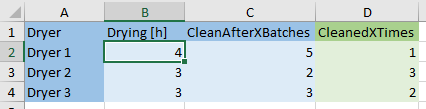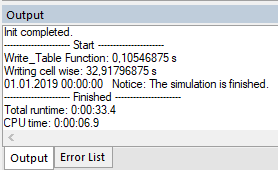•4. October 2019

# Applying Table Objects

Table objects in INOSIM are indexed tables. By the row or column headline, you can directly access a cell’s content without having to search for the right row or column with a For-Loop.To overwrite an operation’s duration in a parameter control with a value from the table, this code might be used:

``````Sub Drying_Duration(cop As OrderOperation, mop As RecipeOperation)
cop.Duration = t_process(cop.Unit.Name, "Drying [h]") * 3600
End Sub``````

To be able to use data from an Excel sheet as a Table object in the simulation, first the data from Excel have to be transferred to the Table object, e.g.,  in the Simulation_Init procedure. In the first part of this tip, the Read_Table function, which you can apply easily in your projects, is introduced. Table objects are also useful to save large amounts of custom results during simulation, e.g., like this:

``````i = t_results.ColumnCount
t_results(cop.Unit.Name,i+1) = cop.OrderProcedure.CustomAttribute("CIP Delay")``````

This Table object, for example, might be written in an End_Sim procedure cell wise, using the CellYX property. A current computer, though, would take 1 s to write 1000 cells. With large amounts of data, long waiting times might result. Hence, in the second part of this tip, the Write_Table function is introduced, by which writing is performed approximately a hundred times faster than the writing of single cells.

The function expects as input parameters

• XLS_Sheet (the string name of the Excel sheet),
• VBA_Table (the Table object defined before),
• rs and cs (first row and first column),
• re and ce (last row and last column, 0 for all),
• ri and ci (activate the row and column index of the Table object). When the row index is activated, the first row is used as index (columns analogously).

For example, in the Simulation_Init procedure use the following code:

``Read_Table("Input", t_input,1,1,0,0,1,1)``

By this code, the complete Excel sheet is read, since the function re = 0 interprets this as “all available rows” (analog for ce). Of course, you may also, from row 2, only read columns 3 to 8 and only enable the row index with Read_Table(“Input”, t_input,2,3,0,8,1,0).

Keep in mind that as no duplicate row or column indices may be created, the row (or column) used as index may not contain duplicate entries.

``````Function Read_Table(XLS_Sheet As String, VBA_Table As Table, rs As Integer, cs As Integer, re As Integer, ce As Integer, ri As Boolean, ci As Boolean)
'Support function for reading excel sheets into VBA table objects

'XLS_Sheet = sheet name of excel sheet
'VBA_Table = table object in VBA
'rs and cs = first row(rs)/first column (cs) to be used in sheet
're and ce = last row(re)/last column (ce) to be used in sheet. re = 0 or ce = 0 results in all rows/columns of sheet to be used
'ri and ci = settings, whether first row/column should be used as an index in table object

Set VBA_Table = New Table
Dim s As Sheet
Set s = Parameters.Sheets(XLS_Sheet)
Dim ro As Integer, co As Integer   'Offset für Zeilen-/Spaltenindex

s.BufferEnabled = True
Dim r,c As Integer
If re = 0 Then
re = s.UsedRows
End If
If ce = 0 Then
ce = s.UsedColumns
End If
If ri Then
'Switch row index on and set offset for first column = 0
VBA_Table.RowIndex = True
co = 0
Else
'Set offset for first column = 0
co = 1
End If
If ci Then
'Switch column index on and set offset for first row = 0
VBA_Table.ColumnIndex = True
ro = 0
Else
'Set offset for first row = 0
ro = 1
End If

'pre dimension the table-object to reduce memory usage for large tables
VBA_Table.ColumnCount = ce-cs
VBA_Table.RowCount = re-rs

'write data from sheet into Table
For r = 0 To re-rs
For c = 0 To ce-cs
If r + ro = 0 Or c + co = 0 Then
If s.CellYX(r+rs,c+cs) <> "" Then
VBA_Table(r+ro,c+co) = CStr(s.CellYX(r+rs,c+cs))
End If
Else
If IsDate(s.CellYX(r+rs,c+cs)) Then
VBA_Table(r+ro,c+co) = CStr(s.CellYX(r+rs,c+cs))
Else
VBA_Table(r+ro,c+co) = s.CellYX(r+rs,c+cs)
End If
End If
Next
Next
End Function``````

## Write_Table function

This function expects as input parameters

• VBA_Table (a Table object with results),
• t_rs and t_cs (first row and first column of the Table object),
• t_re and t_ce (last row and last column of the Table object, 0 for all),
• XLS_Sheet (the string name of the Excel sheet),
• s_rs and s_cs (first row and first column of the sheet to be written).

For example, in the End_Sim procedure use the code:

``Write_Table(t_results,0,0,0,0,"Results",1,1)``

By this code, the complete Table object is written into the sheet t_results. Please keep in mind that the index row or the index column has the number 0. For reading out the row index, 0 is the first row. With t_re = 0, all available rows are selected (analogously for ce). You can of course also write the Table object to Excel without indices and only the columns 3-8 without overwriting the columns 1-3 of the sheets with:

``Write_Table(t_results,1,3,0,8,"Results",1,4)``
``````Function Write_Table(VBA_Table As Table, t_rs As Long, t_cs As Long, t_re As Long, t_ce As Long, XLS_Sheet As String, s_rs As Long, s_cs As Long) ', ri As Boolean, ci As Boolean)
'Support function for writing VBA table objects into an Excel Sheet using RangeYX method

'VBA_Table = VBA table object
't_rs and t_cs = first row(rs)/first column (cs) of table object. Use t_rs = 0 and t_cs = 0 to include the index
't_re and t_ce = last row(re)/last column (ce) of table object. t_re = 0 or t_ce = 0 results in all rows/columns of sheet to be used
'XLS_Sheet = sheet name of excel sheet as string
's_rs and t_cs = first row/first column of the excel sheet to be used

'set re, ce
Dim r As Long, c As Long
If t_re = 0 Then
t_re = VBA_Table.RowCount
End If
If t_ce = 0 Then
t_ce = VBA_Table.ColumnCount
End If

'set array dimensions
Dim a() As Variant
ReDim a(t_re-t_rs,t_ce-t_cs)

'writing into array
For r = t_rs To t_re
For c = t_cs To t_ce
If Not IsNull(VBA_Table(r,c)) Then
a(r-t_rs,c-t_cs) = VBA_Table(r,c)
End If
Next
Next

'writing array to sheet
Dim s As Sheet
Set s = Parameters.Sheets(XLS_Sheet)

'(first row, first column, first row + number of rows, first column + number of columns)
s.RangeYX(s_rs, s_cs, s_rs+t_re-t_rs, s_cs+t_ce-t_cs) = a()

End Function``````

## Demo project

### Experiment General Understanding

In the demo project, in the Simulation_Init Procedure an Excel sheet with 10000 values by the Read_Table Function is transferred into the Table object t_input. During the simulation, the Table object t_results is created and, in the End_Sim procedure, by use of the Write_Table function it is written into the Excel sheet Results. For comparison, t_results is also written cell wise into Excel sheet Results cell wise, and the particularly needed calculation time is written to the console.### Experiment Process Example

Given are 3 dryers with different drying durations and different cleaning demand (after 2-5 batches).

The drying duration in the parameter control Drying_Duration is read out of the Table object t_process and is assigned to the operation.

In the link condition Dryer_Clean, the CustomAttribute BatchCounter is compared with the value CleanAfterXBatches from t_process to be able to decide if the dryer has to be cleaned. When cleaning is processed, the value in cell t_process([dryer name], CleanedXTimes) is counted up by 1.

In the End_Sim procedure, with the Write_Table function the column 3 (CleanedXTimes) is written back to the Excel sheet.

(For registered INOSIM Users only)

• Example Project
• VBA Function Write_Table

## More Questions?

Array ( [posts_per_page] => 3 [post_type] => [category__in] => Array (  => 36 ) [orderby] => rand [order] => ASC )

## More Tips & Tricks

23. February 2021

#### INOSIM Remote Control via the COM-Interface

Did you know INOSIM can be remote-controlled by any program that can send signals via the COM-Interface? Let us sketch a scenario of how this…

22. February 2022

#### Modeling Fluctuating Resource Demands

In the process industry, it is not unlikely that a resource demand changes over the time of a process operation. Typical examples are cooling jackets…

28. May 2021

#### Custom Probability Distributions

Learn how to use the implemented probability distributions in INOSIM to add stochastic failures or process parameters to your model, and how to set up…

Direct Contact